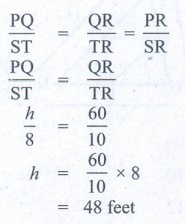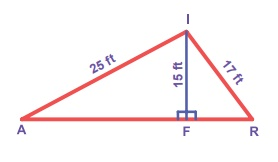Home | | Maths 8th Std | Exercise 5.3

# Exercise 5.3

8th Maths : Chapter 5 : Geometry : Miscellaneous Practice problems, Challenge Problems, Text Book Back Numerical problems, Exercises Questions with Answers, Solution

Exercise 5.3

Miscellaneous Practice Problems

1. In the figure, given that 1 2 and 3 4 . Prove that ∆ MUG TUB.Solution:

Proof:

Statements: Reasons

1. In ΔMUG and ΔTUB

MU = TU : 3 = 4, opposite sides of equal angles

2. UG = UB : 1 = 2

Side opposite to equal angles are equal

3. GUM = BUT : Vertically opposite angle

4. ΔMUG ≡  ΔTUB : SAS criteria By 1, 2 and 3

2. From the figure, prove that ∆SUN ~ ∆RAY.Solution:

Proof: from the ΔSUN and ΔRAY

SU = 10

UN = 12

SN = 14

RA = 5

AY = 6

RY = 7

We haveSU/RA = 10/5 = 2/1    …….(1)

UN/AY = 12/6 = 2/1      …….(2)

SN/RY = 14/7 = 2/1      …….(3)

From (1), and (2) and (3) we have

SU/RA = UN/AY = SN/RY = 2/1The sides are proportional

ΔSUN ~ Δ RAY

3. The height of a tower is measured by a mirror on the ground at R by which the top of the tower’s reflection is seen. Find the height of the tower. If ∆PQR ~ ∆STRSolution:

The image and its reflection make similar shapes

Δ PQR ~ Δ STR

PQ/ST = QR/TR =PR/SR= 48 feet

Height of the tower = 48 feet.

4. Find the length of the support cable required to support the tower with the floor.Solution:

From the figure, by Pythagoras theorem,

x2 = 202 + 152

= 400 + 225 = 625

x2 = 252 x = 25ft.

The length of the support cable required to support the tower with the floor is 25 ft.

5. Rithika buys an LED TV which has a 25 inches screen. If its height is 7 inches, how wide is the screen? Her TV cabinet is 20 inches wide. Will the TV fit into the cabinet? Give reason.

Solution:

Take the sides of a right angled triangle ΔABC as

a = 7 inches

b = 25 inches

c = ?By Pythagoras theorem,

b2 = a2 + c2

252 = 72 + c2

c2 = 252 72 = 625 − 49 = 576

c2 = 242

=> c = 24 inches

Width of TV cabinet is 20 inches which is lesser than the width of the screen ie.24 inches.

The TV will not fit into the cabinet.

Challenging problems

6. In the figure, TMA IAM and TAM IMA . P is the midpoint of MI and N is the midpoint of AI. Prove that ∆ PIN ~ ∆ ATM.Solution:

Proof:

Statements: Reasons

1. TMA = IAM

TAM = IMA  : Reasons: given

2. ATM = TIM : Reasons: Remaining angle

By angle sum property

3. IP = PM = IP/PM = 1

In = NA = IN/NA = 1 : Reasons: P is the midpoint of IM and N is the midpoint of IA

4. IP / PM = IN / NA  :  Reasons: By 3

5. PN || MA : Reasons: By 4

6. IPN = IMN

INP = IAM   :   Reasons: By 5

7. In ΔPIN and ΔATM

(i) IPN = TAM

(ii) INP = TMA

(iii) ATM = PIN  : Reasons: By 1, 2 and 6

8. ΔPIN ~ ΔATM   :  Reasons: By AAA criteria

7. In the figure, if FEG 1 then, prove that DG 2 = DE . DF .Solution:

Proof:

Statements : Reasons

1. FEG ≡ 1

⇒ ∠DEG = 180° − FEG

Reasons:

Given

linear pair

2. FDG + DFG = 1

EDG + DFG = 1

Reasons: Exterior angle = Sum of interior opposite angles

∵ ∠FDG = EDG

3. DEG = 180° − FEG

DEG = 180 − 1

Reasons: By 1

4. In ΔDFG

DGF =180° − [FDG + DFG]

Reasons: Angle sum property

5. DGF= 180° − 1

Reasons: By 2

6. DGF = DEG

Reasons: By 3

7. EDG = EDG

Reasons: Common in ΔFDG and ΔEDG

8. ∴ ∠DGE = DFG

Reasons: Remaining angle by angle sum property and by 6

9.   ΔDGF ~ ΔDEG

Reasons: By 6, 7, 8

By AAA similarity

10. DG / DE = GF /EG = DF / DG

Reasons: Corresponding sides of similar trianlge are proportional.

11. DG / DE = DF / DG

DG. DG = DF. DE

DG2 = DE.DF

Reasons: From 9

8. The diagonals of the rhombus is 12 cm and 16 cm. Find its perimeter. (Hint: the diagonals of rhombus bisect each other at right angles).

Solution:

Here AO = CO = 8 cm

BO = DO = 6 cm

(  the diagonals of rhombus bisect each other at right angles)In Δ AOB, AB2 = AO2 + OB2

= 82 + 62 = 64 + 36

= 100 = 102

AB = 10

Since it is a rhombus, all the four sides are equal.

AB = BC = CD = DA

Its Perimeter = 10 + 10 + 10 + 10 = 40 cm

9. In the figure, find AR.Solution:

Δ AFI, Δ FRI are right triangles.

By Pythagoras theorem,

AF2 = AI2 − FI2

= 252 − 152

= 625 − 225 = 400 = 202

AF = 20 ft.     ….(1)

FR2 = RI2 − FI2

= 172 − 152 = 289 – 225 = 64 = 82

FR = 8 ft.

AR = AF + FR

= 20 + 8 = 28 ft.

10. In DEF, DN, EO, FM are medians and point P is the centroid. Find the following.

(i) IF DE = 44, then DM = ?

(ii) IF PD = 12, then PN = ?

(iii) If DO = 8, then FD = ?

(iv) IF OE = 36 then EP = ?Solution:

Given DN, EO, FM are medians.

FN = EN

DO = FO

EM = DM

(i) If DE = 44, then

DM = 44 / 2 = 22

DM = 22

(ii) If PD = 12, PN = ?

PD / PN = 2/1

12 / PN = 2/1 PN = 12/2 = 6.

PN = 6

(iii) If DO = 8, then

FD = DO + OF

= 8 + 8

FD = 16

(iv) IF OE = 36,

then EP / PO = 2 / 1

EP / 2 = PO

OE = OP + PE

36 = [PE / 2] + PE36 = [PE / 2] +  [2PE / 2]

36 = 3PE / 2

PE = [36 × 2] / 3

PE = 24

Exercise 5.3

Miscellaneous Practice Problems

3. 48 ft

4. 25 ft

5. No, the wide of cabinet is lesser than the wide of TV

Challenging Problems

8. 40cm

9. 28 ft

10. (i) 24 (ii) 6 (iii) 16 (iv) 24

Tags : Questions with Answers, Solution | Geometry | Chapter 5 | 8th Maths , 8th Maths : Chapter 5 : Geometry
Study Material, Lecturing Notes, Assignment, Reference, Wiki description explanation, brief detail
8th Maths : Chapter 5 : Geometry : Exercise 5.3 | Questions with Answers, Solution | Geometry | Chapter 5 | 8th Maths